CiteScore 2.0
More on impact ›

# Frontiers in Applied Mathematicsand Statistics

## ORIGINAL RESEARCH article

Front. Appl. Math. Stat., 11 May 2015 | https://doi.org/10.3389/fams.2015.00004

# Generic convergence of infinite products of nonexpansive mappings with unbounded domains

• Department of Mathematics, Technion – Israel Institute of Technology, Haifa, Israel

We study the generic convergence of infinite products of nonexpansive mappings with unbounded domains in hyperbolic metric spaces.

## 1. Introduction and the Main Result

Let (X, ρ) be a metric space and let R1 denote the real line. We say that a mapping c : R1X is a metric embedding of R1 into X if ρ(c(s), c(t)) = |st| for all real s and t. The image of R1 under a metric embedding will be called a metric line. The image of a real interval [a, b] = {tR1: atb} under such a mapping will be called a metric segment.

Assume that (X, ρ) contains a family M of metric lines such that for each pair of distinct points x and y in X, there is a unique metric line in M which passes through x and y. This metric line determines a unique metric segment joining x and y. We denote this segment by [x, y]. For each 0 ≤ t ≤ 1, there is a unique point z in [x, y] such that

This point is denoted by (1 − t)xty. We say that X, or more precisely, (X, ρ, M), is a hyperbolic metric space if

$ρ(12x⊕12y,12x⊕12z)≤12ρ(y,z)$

for all x, y, and z in X. An equivalent requirement is that

$ρ(12x⊕12y,12w⊕12z)≤12(ρ(x,w)+ρ(y,z))$

for all x, y, z, and w in X. A set KX is called ρ-convex if [x, y] ⊂ K for all x and y in K.

It is clear that all normed linear spaces are hyperbolic in this sense. A discussion of more examples of hyperbolic spaces and, in particular, of the Hilbert ball can be found, for example, in Goebel and Reich  and Reich and Shafrir .

Let (X, ρ, M) be a complete hyperbolic metric space, and let KX be a nonempty, closed and ρ-convex subset of (X, ρ). For each C: KK, set C0(x) = x for all xK. Denote by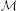the set of all sequences {At}t = 1 of mappings At : KK, t = 1, 2, …, such that for all integers t ≥ 1,

For each xX and each r > 0, set

B(x, r) = {yX: ρ(x, y) ≤ r} and BK(x, r) = B(x, r) ∩ K.

Fix θ ∈ K. For each M, ϵ > 0, set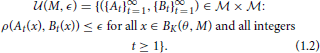We equip the setwith the uniformity which has the base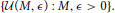It is not difficult to see that the uniform spaceis metrizable (by a metric d) and complete.

Denote by* the set of all {At}t = 1for which there exists a point $\stackrel{{˜}}{{x}}$K satisfying

Denote by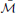* the closure of the set* in the uniform space. We consider the topological subspace*equipped with the relative topology and the metric d.

In this paper we study the asymptotic behavior of (unrestricted) infinite products of generic sequences of mappings belonging to the space* and obtain convergence to a unique common fixed point. More precisely, we establish the following result, which generalizes the corresponding result in Reich and Zaslavski  (see also  and ). That result was obtained in the case where the set K was bounded.

Theorem 1.1. There exists a set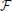* which is a countable intersection of open and everywhere dense subsets of the complete metric space (*, d) such that for each {Bt}t = 1, the following properties hold:

(a) there exists a unique point xK such that Bt(x) = x for all integers t ≥ 1;

(b) if t ≥ 1 is an integer and yK satisfies Bt(y) = y, then y = x;

(c) for each ϵ > 0 and each M > 0, there exist a number δ > 0 and a neighborhood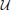of {Bt}t = 1 in the metric space* such that if {Ct}t = 1, t ∈ {1, 2, …}, and if yBK(θ, M) satisfies ρ(y, Ct(y)) ≤ δ, then ρ(y, x) ≤ ϵ;

(d) for each ϵ > 0 and each M > 0, there exist a neighborhoodof {Bt}t = 1 in the metric space*, a number δ > 0 and a natural number q such that if {Ct}t = 1, mq is an integer, r: {1, …, m} → {1, 2, …}, and if {xi}mi = 0K satisfies

$ρ(x0,θ)≤M$

and

$ρ(Cr(i)(xi − 1),xi)≤δ, i=1,…,m,$

then

$ρ(xi,x¯)≤ϵ, i=q,…,m.$

## 2. Proof of Theorem 1.1

Elements of the spacewill occasionally be denoted by a boldface letters: A = {At}t = 1, B = {Bt}t = 1, C = {Ct}t = 1, respectively.

Let A = {At}t = 1* and γ ∈ (0, 1). There exists a point xAK such that

For each integer t ≥ 1 and each xK, set

By (1.1), (2.1), and (2.2), for all integers t ≥ 1 and all points x, yK,

and

In view of (2.2–2.4),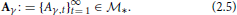Let n be a natural number. Fix a number

a number

a positive number

and an integer

There exists an open neighborhood V(A, γ, n) of {Aγ, t}t = 1 in* such that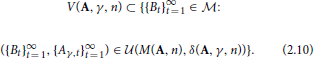Assume that

is an integer,

and that a sequence {xi}mi = 0K satisfies

and

We now show by induction that for all integers i = 0, …, m,

and if i < m, then

Assume that p ∈ {0, …, m − 1}, (2.16) and (2.17) hold for all i = 0, …, p and that (2.18) holds for all nonnegative integers i < p. [Note that in view of (2.6), (2.7), and (2.14), our assumption holds for p = 0]. It follows from (2.3), (2.4), and (2.15) that

By (2.17), which holds for i = p, (1.2), (2.10), and (2.11),

Relations (2.19) and (2.20) imply that

Thus, (2.18) holds for i = p. It follows from (2.16), which holds for i = p, (2.6), (2.8), and (2.21) that

$ρ(xp + 1,xA)≤(1−γ)r(A,n)+2δ(A,γ,n) ≤(1−γ)r(A,n)+2−1γ≤r(A,n).$

By the above relation and (2.7),

$ρ(xp + 1,θ)≤ρ(xp + 1,xA)+ρ(xA,θ) ≤r(A,n)+ρ(xA,θ)≤M(A,n).$

Hence (2.16) and (2.17) hold for i = p + 1 and the assumption made for p also holds for p + 1. Therefore, our assumptions hold for p = m, (2.16) and (2.17) hold for all i = 0, …, m, and (2.18) holds for all i = 0, …, m − 1.

We claim that for all i = q(A, γ, n), …, m,

First we show that there exists i ∈ {0, …, q(A, γ, n)} such that (2.22) holds.

Assume the contrary. Then

By (2.8), (2.18), and (2.23), for all integers i = 0, …, q(A, γ, n) − 1,

$ρ(xi,xA)−ρ(xi + 1,xA)≥γρ(xi,xA)−2δ(A,γ,n)≥γn−1−2δ(A,γ,n)≥γ(2n)−1.$

In view of the above inequality and (2.16),

$r(A,n)≥ρ(x0,xA)≥ρ(x0,xA)−ρ(xq(A,γ,n),xA)=∑i = 0q(A,γ,n)−1(ρ(xi,xA)−ρ(xi + 1,xA))≥q(A,γ,n)γ(2n)−1$

and so,

$q(A,γ,n)≤2nr(A,n)γ−1.$

This contradicts (2.9). The contradiction we have reached proves that there indeed exists an integer j ∈ {0, …, q(A, γ, n)} such that

Next we claim that (2.2) holds for all integers i ∈ {j, …, m}.

Indeed, by (2.24), inequality (2.22) is true for i = j. Now assume that i ∈ {j, …, m}, i < m and (2.22) holds. There are two cases:

Assume now that (2.25) holds. In view of (2.8), (2.18), and (2.25),

$ρ(xi + 1,xA)≤(1−γ)ρ(xi,xA)+2δ(A,γ,n) ≤(2n)−1+2δ(A,γ,n)≤n−1.$

Assume that (2.26) holds. Then it follows from (2.8), (2.18), (2.22), and (2.26) that

$ρ(xi + 1,xA)≤(1−γ)ρ(xi,xA)+2δ(A,γ,n) =ρ(xi,xA)−γρ(xi,xA) +2δ(A,γ,n) ≤n−1−γ(2n)−1+2δ(A,γ,n)≤n−1.$

Thus, in both cases,

$ρ(xi + 1,xA)≤n−1.$

This means that we have shown by induction that (2.22) is indeed valid for all i = q(A, γ, n), …, m. Clearly, we have proved that the following property holds:

(P) For each

${Ct}t = 1∞∈V(A,γ,n),$

each integer mq(A, γ, n), each

$r:{1,…,m}→{1,2,…}$

and each sequence {xi}mi = 0K which satisfies

$ρ(x0,θ)≤n$

and

$ρ(Cr(i)(xi − 1),xi)≤δ(A,γ,n), i=1,…,m,$

we have

$ρ(xi,xA)≤n−1, i=q(A,γ,n),…,m.$

Set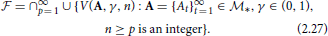By (1.1), (2.1), and (2.2), for each A = {At}t = 1*, each γ ∈ (0, 1), each integer t ≥ 1 and each xK, we have

In view of (1.2) and (2.28),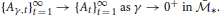When combined with (2.27), this implies thatis a countable intersection of open and everywhere dense subsets of*.

Assume that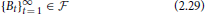and M, ϵ > 0. Choose a natural number p such that

By (2.27) and (2.29), there exist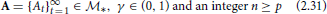such that

Let

let t ≥ 1 be an integer and consider the sequence {Bit(x)}i = 0. By (2.30)–(2.33) and property (P) (applied to {Cs}s = 1 = {Bs}s = 1 and r(j) = t, j = 1, 2, …), for all integers iq(A, γ, n), we have

Since ϵ is an arbitrary positive number, we conclude that for each point zBK(θ, M) and each integer t ≥ 1, {Bit(z)}i = 0 is a Cauchy sequence. Since M is any positive number, we see that for each integer t ≥ 1 and each zK, there exists

$limi→∞Bti(z)$

in (X, ρ). In view of (3.34), for every integer t ≥ 1 and every zBK(θ, M),

$ρ(limi→∞Bti(z),xA)≤ϵ.$

This implies that for each pair of points z1, z2BK(θ, M) and for each pair of natural numbers t1, t2,

$ρ(limi→∞Bt1i(z1),limi→∞Bt2i(z2))≤2ϵ.$

Since ϵ, M are arbitrary positive numbers, we may conclude that for each pair of integers t1, t2 ≥ 1 and each pair of points z1, z2K,

$limi→∞Bt1i(z1)=limi→∞Bt2i(z2).$

Let xK be such that

In view of (2.35),

It immediately follows from (2.35) and (2.36) that properties (a) and (b) hold. We claim that property (c) also holds.

Let

${Ct}t = 1∞∈V(A,γ,n), t∈{1,2,…,}, y∈BK(θ,M)$

and assume that

Set

It follows from (2.37) and (2.38) that for all integers t ≥ 1,

By (2.30), (2.31), (2.37–2.39) and property (P) applied to any integer mq(A, γ, n) and xi = yi, i = 0, …, m,

$ρ(yi,xA)≤n−1, i=q(A,γ,n),…,m,$

and

In view of (2.30), (2.31), (2.34), (2.35), and (2.40),

Thus, property (c) does hold, as claimed.

Finally, we show that property (d) holds too. It follows from (2.34) and (2.35) that

$ρ(xA,x¯)≤n−1.$

Assume that

${Ct}t = 1∞∈V(A,γ,n),$

let mq(A, γ, n) be an integer, r: {1, …, m} → {1, 2, …}, and let {xi}mi = 0K satisfy

$ρ(x0,θ)≤M$

and

$ρ(Cr(i)(xi − 1),xi)≤δ(A,γ,n), i=1,…,m.$

By the relations above and property (P),

It now follows from (2.30), (2.31), (2.41), and (2.42) that for all integers i = q(A, γ, n), …, m,

$ρ(xi,x¯)≤ρ(xi,xA)+ρ(xA,x¯)≤2n−1<ϵ.$

Thus, property (d) indeed holds. This completes the proof of Theorem 1.1.

## Conflict of Interest Statement

The authors declare that the research was conducted in the absence of any commercial or financial relationships that could be construed as a potential conflict of interest.

## Acknowledgments

SR was partially supported by the Israel Science Foundation (Grant No. 389/12), by the Fund for the Promotion of Research at the Technion and by the Technion General Research Fund.

## References

1. Goebel K, Reich S. Uniform Convexity, Hyperbolic Geometry, and Nonexpansive Mappings. New York, NY; Basel: Marcel Dekker (1984).

2. Reich S, Shafrir I. Nonexpansive iterations in hyperbolic spaces. Nonlin Anal. (1990) 15:537–58.

3. Reich S, Zaslavski AJ. Convergence of generic infinite products of nonexpansive and uniformly continuous operators. Nonlin Anal. (1999) 36:1049–65.

4. Reich S, Zaslavski AJ. Inexact powers and infinite products of nonlinear operators. Int J Math Stat. (2010) 6:89–109.

5. Reich S, Zaslavski AJ. Genericity in Nonlinear Analysis, Developments in Mathematics, Vol. 34. New York, NY: Springer (2014).

Keywords: fixed point, generic property, hyperbolic metric space, infinite product, nonexpansive mapping

Citation: Reich S and Zaslavski AJ (2015) Generic convergence of infinite products of nonexpansive mappings with unbounded domains. Front. Appl. Math. Stat. 1:4. doi: 10.3389/fams.2015.00004

Received: 20 March 2015; Accepted: 10 April 2015;
Published: 11 May 2015.

Edited by:

Jin Liang, Shanghai Jiao Tong University, China

Reviewed by:

Ming Tian, Civil Aviation University of China, China
Yekini Shehu, University of Nigeria, Nigeria

Copyright © 2015 Reich and Zaslavski. This is an open-access article distributed under the terms of the Creative Commons Attribution License (CC BY). The use, distribution or reproduction in other forums is permitted, provided the original author(s) or licensor are credited and that the original publication in this journal is cited, in accordance with accepted academic practice. No use, distribution or reproduction is permitted which does not comply with these terms.

*Correspondence: Alexander J. Zaslavski, Department of Mathematics, Technion – Israel Institute of Technology, Amado Mathematics Building, Haifa 32000, Israel, ajzasl@techunix.technion.ac.il